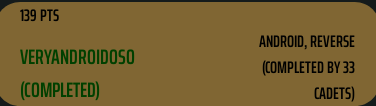# DEF CON Quals 2019 : VERYANDROIDOSO

Here is my writeup for `VERYANDROIDOSO` task. Ofcourse with frida :DApp takes input from us and checks if it is correct flag. Length of flag should be 23 enclosed with OOO{..}. Also inside of brackets only hex [0-9a-f].
18(23-5) divided into 9 part with each part length of 2.

## Solverrr

In solver class there are `solver`, `getSecretNumber`, `scramble`(not really), `sleep (!)`:
and exported functions from native-lib:

`m0, m1, m2, m3, m4, m6, m7, m8, m9`

• `getSecretNumber` gets one byte from hash of certificate of application. So its always returns same value to same input.

• `sleep` function takes input, and sleep nanosecond*input and returns how much nanosecond is passed (which can be vary).

• `scramble` does following :

Looks like it depends on output of sleep function ?

Nope :D because sqrt(4sleep^2)/sleep always returns 2. So overall scramble is same as `return (i+2+321)%256`
Same as `getSecretNumber` this function is always returns same value to same input.

• If you open up ghidra and decompile you can see that m0-m1-m2-m3-m4-m6 doesn’t depend on any value other than its inputs. But
m7 depends on s1 and s2. m8 depends on s3 and s4. If you look at offset of s4, you can see it is not defined. m9 takes one input and `CREATES` s4 data.
So m9 is very important to m8’s operations and output. s1, s2 and s3 is already defined in binary and never changed with any other function.

Now comes fun part.

## Fridaa

in solve function there are huge if blocks for each input part.

For i1 code is as follows :

To not return false, we need to find correct m0 value . which is `m0 & 255 == 172` . iff m0 = 172 . As you can see m0 set with output of m0 function.
So we need to find correct first parameter to m0. How ? frida :D

How did I know second parameter of m0 ?. Lets just give random input : `OOO{123456789012345678}`

Attach with `frida -U --no-pause -f ooo.defcon2019.quals.veryandroidoso -l 1.txt`

Then frida outputs :

```Scramble 80 13 Secret 113 80```

secret function output is 113.

Then I bruteforced all values for m0 in for loop. Frida says m0 should be 53. 53 is input to m0. And we have just 1 option since we ANDed with 255.
If you search `m0(53,` in jadx you can find i1 == 250. Our first byte is 0xfa . If it is correct then We must see second scramble output in frida.

Try `OOO{fa3456789012345678}`

Same script gives:

```Scramble 80 13 Secret 113 80 Scramble 147 80 Secret 201 147```

So it is correct !

For i2 AND

Our condition is m0 & 255 == 6, m0 should be 6. You can find correct secret value same as in step 1. So our for new for loop is :

Add it to frida script. Then output is :
`m0 = 53,m1 = 35` => i2 = 180

[250,180]

So far so good.

For i3 :

This time output is ANDed with 251. i10 & 251 == 146 gives two option.

m2 = 7 and m2 = 11 ==> 254 and 52

.
.
.

Overall dc.txt gives :

(Careful here, you should find correct i1 for m0) But since there is no m5 and it just checks over itself you can assign it as correct input.

We have 2*2*16*64 different combination for now.

## Bigger plan

After m7 if block, m9(i1+..+i7*i8) is called. This will change s4. Then ONE more if block comes for last hex. Since m8 is dependent on s4 we CANNOT bruteforce like before.

If block :

i10 must be 103.

To find last hex : frida script:

``````Iterate over possible inputs
Clear s4.
Calculate input for m9
Call m9
Find correct value for m8
Store solution
``````

Frida can easily handle memory. Calculate all possible values : calc.py

Here t is possible values. Script will find correct input to m8 function. Then you will need to find correct i8. I found older inputs with manual search, but for this case I used python

Now there is just last check !

This time we have all inputs lets just give it to solve function.

Last script

Bypass sleep function so we wont wait.

## Fl4g

Now enter something like `OOO{123456789012345678}` and wait until frida finds correct value ! :DFlag is hex values of those integers:
`OOO{fab43416484944beba}`